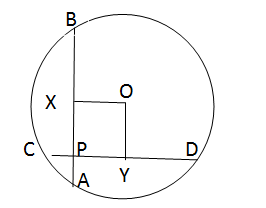Two mutually perpen...

Share:
Clear all

# Two mutually perpendicular chords AB and CD intersect at P. AP = 4, PB = 6, CP = 3. Find radius of the circle.

2 Posts
2 Users
0 Likes
1,622 Views(@soham)
Posts: 131
Estimable Member
Topic starter

Two mutually perpendicular chords AB and CD intersect at P. AP = 4, PB = 6, CP = 3. Find the radius of the circle.

1. 31.25(1/2)
2. 37.5(1/2)
3. 26(1/2)
4. 52(1/2)
Posted : 23/03/2021 8:34 pm(@rahul)
Posts: 825When 2 chords AB and CD intersect at P then AP * PB = CP * PD
Hence 4 * 6 = 3 * PD
Thus, PD = 8
Now AB = AP + PB = 10
And CD = CP + PD
Thus, CD = 11
Consider the circle with center O.
Drop a perpendicular from O to chord AB and CD.
This will bisect the chords at X and Y i.e AX=XB and CY = YD.
Here AX = AP + PX i.e 5 = 4 + PX
PX = 1
Similarly, since CD = 11, PY+CP+YD = 11,
=> PY = 11-3-5.5 = 2.5.
PY = OX and PX = OY.
So, PXOY will from a rectangle as seen in the figure.
Now consider the triangle BOX, it is a right triangle where OB is the radius.
XB = 5, OX = 2.5
Then OB = ( OX2 + XB2 )1/2 OB = 31.251/2 Thus radius = 31.251/2 The radius can also be found out using the triangle YOD.

The question is "Find radius of the circle."

##### Hence, the answer is 31.251/2.
Posted : 23/03/2021 9:05 pm
Share: